Test: Boolean Algebra & Minimization Techniques- 2

# Test: Boolean Algebra & Minimization Techniques- 2

Test Description

## 20 Questions MCQ Test Topicwise Question Bank for Electrical Engineering | Test: Boolean Algebra & Minimization Techniques- 2

Test: Boolean Algebra & Minimization Techniques- 2 for Electrical Engineering (EE) 2023 is part of Topicwise Question Bank for Electrical Engineering preparation. The Test: Boolean Algebra & Minimization Techniques- 2 questions and answers have been prepared according to the Electrical Engineering (EE) exam syllabus.The Test: Boolean Algebra & Minimization Techniques- 2 MCQs are made for Electrical Engineering (EE) 2023 Exam. Find important definitions, questions, notes, meanings, examples, exercises, MCQs and online tests for Test: Boolean Algebra & Minimization Techniques- 2 below.
Solutions of Test: Boolean Algebra & Minimization Techniques- 2 questions in English are available as part of our Topicwise Question Bank for Electrical Engineering for Electrical Engineering (EE) & Test: Boolean Algebra & Minimization Techniques- 2 solutions in Hindi for Topicwise Question Bank for Electrical Engineering course. Download more important topics, notes, lectures and mock test series for Electrical Engineering (EE) Exam by signing up for free. Attempt Test: Boolean Algebra & Minimization Techniques- 2 | 20 questions in 60 minutes | Mock test for Electrical Engineering (EE) preparation | Free important questions MCQ to study Topicwise Question Bank for Electrical Engineering for Electrical Engineering (EE) Exam | Download free PDF with solutions
 1 Crore+ students have signed up on EduRev. Have you?
Test: Boolean Algebra & Minimization Techniques- 2 - Question 1

### Consider the following statements associated with Boolean algebra: 1. There is nothing like subtraction or division in Boolean algebra. 2. There is possibility of having negative or fractional numbers. 3. A variable has no numerical significance. 4. Logical multiplication is same as the AND operation, and logical addition is the same as the OR operation. 5. There are only three constants within the Boolean system. Which of the statements given above are correct?

Detailed Solution for Test: Boolean Algebra & Minimization Techniques- 2 - Question 1

Statement 2 and 5 are not correct because there is no possibility of having negative and fractional numbers is Boolean algebra. Also, there are only two constant 0 and 1 (don’t care (x) is not a constant) within the Boolean system.

Test: Boolean Algebra & Minimization Techniques- 2 - Question 2

### Assertion (A): Boolean algebra and Binary number system are different from each other. Reason (R): There are some basic operations like AND, OR and NOT which are performed in Boolean algebra.

Detailed Solution for Test: Boolean Algebra & Minimization Techniques- 2 - Question 2

Boolean algebra and Binary number system are different from each other because in Boolean algebra
1 + 1 = 1 while in the binary number system 1 + 1 = 10. There are lot of other examples to prove this.
Reason is also a correct statement because AND, OR and NOT are the three basic operations which are used in Boolean algebra. However, reason is not the correct explanation of assertion.

Test: Boolean Algebra & Minimization Techniques- 2 - Question 3

### According to De Morgan’s theorem: 1. the NOR gate is equivalent to a bubbled NAND gate. 2. individual variables can be removed form under a NOT sign, and a sum of product from can be transformed to a product-of- sums form. 3. the complement of the product of variables is equal to the sum of their individual complements. 4. the NAND gate is equivalent to a bubbled NOR gate. 5. individual variables can be removed from under a NOT sign, and a product-of-sum form can be transformed to a sum-of-products form. Q. Which of the above statements about De Morgan’s theorem is/are not correct?

Detailed Solution for Test: Boolean Algebra & Minimization Techniques- 2 - Question 3
•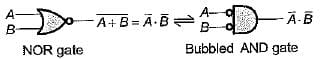Hence, statement-1 is not correct
•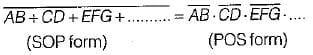Hence, statement-2 is correct.
•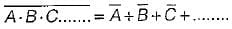Hence, staement-3 is correct.
•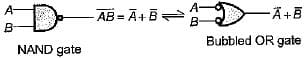Hence, statement-4 is not correct.
•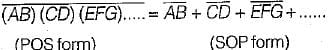Hence, statement 5 is correct.
Test: Boolean Algebra & Minimization Techniques- 2 - Question 4

The complement of the expression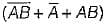is equal to

Detailed Solution for Test: Boolean Algebra & Minimization Techniques- 2 - Question 4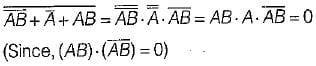Test: Boolean Algebra & Minimization Techniques- 2 - Question 5

Match List-l (Boolean expressions)with List-ll (Minimized expression) and select the correct answer using the codes given below the lists:
List-l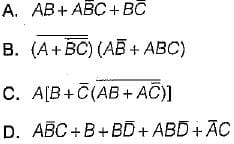List-II
1. 0
2. B + C
3. AC +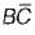4. AB
Codes:
A B C D
(a) 3 4 1 2
(b) 1 2 3 4
(c) 3 1 4 2
(d) 2 3 4 1

Detailed Solution for Test: Boolean Algebra & Minimization Techniques- 2 - Question 5
•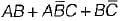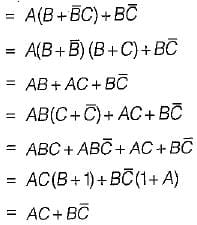•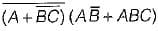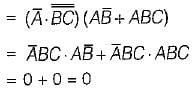•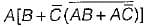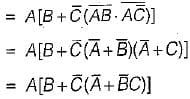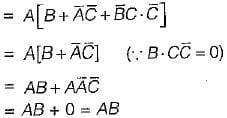•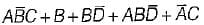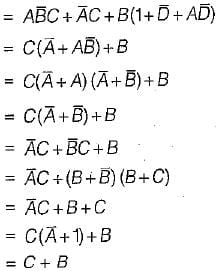Test: Boolean Algebra & Minimization Techniques- 2 - Question 6

The minimized Boolean expression for the logic diagram shown below is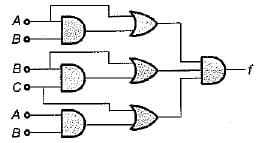Detailed Solution for Test: Boolean Algebra & Minimization Techniques- 2 - Question 6

From given logic diagram,
f = (A+AB) (B + BC) (C + AB)
= A (1 + B).B(1 + C) (C + AB)
= AB (C + AB)
= ABC + AB.AB
= ABC + AB
= AB (C+1) = AB

Test: Boolean Algebra & Minimization Techniques- 2 - Question 7

Assertion (A): The use of K-maps is usually limited to six-variables.
Reason (R): A K-map can not be used for problems involving more than six variables.

Detailed Solution for Test: Boolean Algebra & Minimization Techniques- 2 - Question 7

A K-map map can be used for problems involving any number of variables. But, for more than six variables it becomes tedious to solve the problem sign K-map. Hence, the use oi K-map is usually limited to six-variables. Thus, reason is a false statement.

Test: Boolean Algebra & Minimization Techniques- 2 - Question 8

Assertion (A): The binary number designations of the rows and columns of the K-map are in Gray code.
Reason (R): The Gray code designation is used in K-map to ensure that two physically adjacent squares are really adjacent i.e. their minterms or maxterms differ by only one variable.

Test: Boolean Algebra & Minimization Techniques- 2 - Question 9

Consider the following statements:
1. The terms which cannot be combined, further in the tabular method are called “prime implicants.’’
2. The implicants which will definitely occur in the final expression are called “essential prime implicants.”
3. The prime implicant chart is a pictorial representation of the relationships between the prime implicants and the minterms of the expression.
Which of the above statements is/are correct?

Test: Boolean Algebra & Minimization Techniques- 2 - Question 10

X8X4X2Xis an 8421 BCD inputto a logic circuit whose output is 1 when X8 = 0, X4 = 0 and X2 = 1, or when X8 = 0 and X4 = 1. The simplest output of this logic circuit would be:

Detailed Solution for Test: Boolean Algebra & Minimization Techniques- 2 - Question 10

Denoting the non-complemented variable by 1 and the complemented variable by 0, the equation for the output is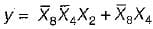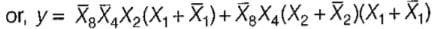= 0011 + 0010 + 0100 + 0101 + 0110 + 0111
= ∑M (2, 3, 4, 5, 6, 7)
The input is a 4-bit BCD, therefore there will be 6 invalid combinations (10,11,1.2,13,14,15) and the corresponding outputs are don’t cares.
Hence, the Boolean equation will be
y = ∑m ( 2, 3, 4, 5, 6, 7) + d(10, 11, 12, 13, 14, 15)
The K-map for the above Boolean equation is shown below: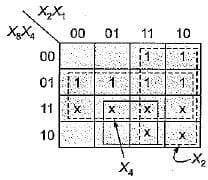From the above K-map, the simplex output of the given logic circuit will be: y = X2 + X4

Test: Boolean Algebra & Minimization Techniques- 2 - Question 11

The inputs to a computer circuit are 4-bits of the binary number A3A2A1A0. The circuit is required to produce 1 if and only if ail of the following conditions hold.
1. The MSB is 1 or any of the other bits are 0.
2. A2 is 1 or any of the other bits are 0.
3. Any of the 4-bits are 0.
The minimized expression for the output of the computer circuit is

Detailed Solution for Test: Boolean Algebra & Minimization Techniques- 2 - Question 11

From the given statements, the Boolean expression must be in the POS form given by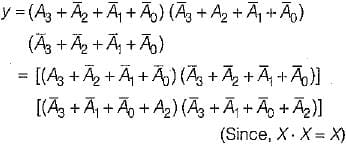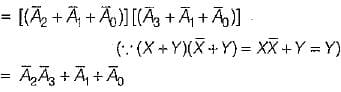Test: Boolean Algebra & Minimization Techniques- 2 - Question 12

The minimum number of gate inputs required to implement the minimized output of the expression given by f are:
f = ∑m (1 ,5 , 6 , 12 , 13 , 14) + d(2, 4)

Detailed Solution for Test: Boolean Algebra & Minimization Techniques- 2 - Question 12

Given, f = ∑m (1 ,5 ,6 ,12 ,13 ,14) + d(2 ,4) (In SOP form)
= πM (0, 3, 7, 8, 9, 10, 11, 15)-d(2, 4) (In POS form)
The K-map for SOP and POS expressions of output are shown below.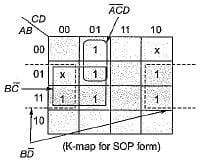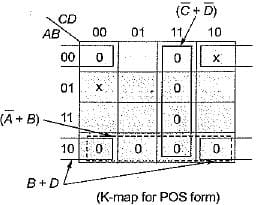In SOP form output is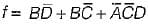In POS form output is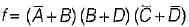The circuit realization for output fusing SOP and POS forms respectively are shown below: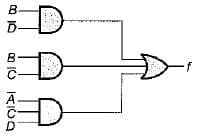(Using SOP output)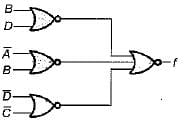(Using POS output)
We see that total number of gate inputs required using SOP output is 10 while that for POS output is 9. Hence, minimum number of inputs required to implement the minimized expression of output is 9 (using POS form).

Test: Boolean Algebra & Minimization Techniques- 2 - Question 13

The minimized expression for the output given by: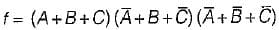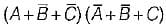Detailed Solution for Test: Boolean Algebra & Minimization Techniques- 2 - Question 13

Given, f =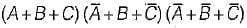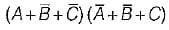The max terms are:
000, 101, 111, 011 ,110
= πM(0, 3, 5, 6, 7)
= ∑m(1, 2, 4)
The K-map is shown below.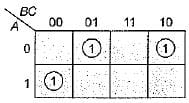Hence, output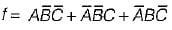Test: Boolean Algebra & Minimization Techniques- 2 - Question 14

For 4 number of variables, maximum possible self dual expressions are

Detailed Solution for Test: Boolean Algebra & Minimization Techniques- 2 - Question 14

For n number of variables, maximum possible self dual expressions are 22n-1
For n = 4, maximum self dual expressions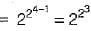= 28 = 256

Test: Boolean Algebra & Minimization Techniques- 2 - Question 15

For the given Venn diagram, the minimized expression for the shaded area is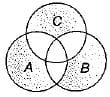Detailed Solution for Test: Boolean Algebra & Minimization Techniques- 2 - Question 15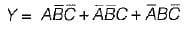Test: Boolean Algebra & Minimization Techniques- 2 - Question 16

For the digital circuit shown below, the output is true if majority number of inputs are false.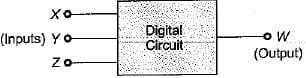The Boolean expression for the output is given by:

Detailed Solution for Test: Boolean Algebra & Minimization Techniques- 2 - Question 16

The truth table for the input-output is shown below.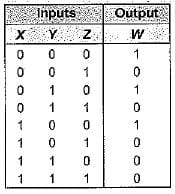Thus, output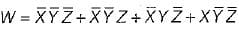Test: Boolean Algebra & Minimization Techniques- 2 - Question 17

Match List-l (Logic circuit) with List-li (Output) and select the correct answer using the codes given below the lists:
List-I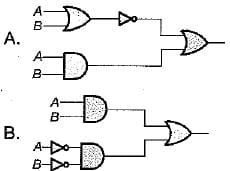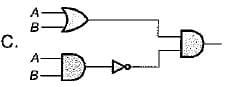List-II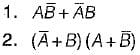3. AB
Codes:
A B C
(a) 3 3 2
(b) 1 2 1
(c) 2 1 3
(d) 2 2 1

Detailed Solution for Test: Boolean Algebra & Minimization Techniques- 2 - Question 17

For logic circuit-A, output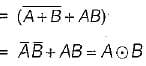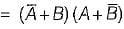For logic circuit-B, output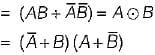For logic circuit-C, output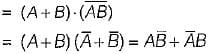Test: Boolean Algebra & Minimization Techniques- 2 - Question 18

Match List-I (Boolean expression) with List-ll (Minimized expression) and select the correct answer using the codes given below the lists:
List-I
A. A. (A + B)
B. AB + BC +C.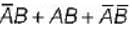D. A +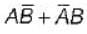List-II
1. A + B
2. AB + C
3. A
4.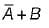Codes:
A B C D
(a) 3 2 4 1
(b) 2 4 3 1
(c) 1 2 3 4
(d) 3 4 1 2

Detailed Solution for Test: Boolean Algebra & Minimization Techniques- 2 - Question 18
• A (A + B) = AA + AB = A + AB
= A(1 + B) = A
•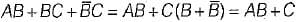•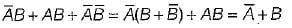•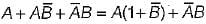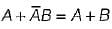Test: Boolean Algebra & Minimization Techniques- 2 - Question 19

The minimized output equation of the K-map shown below has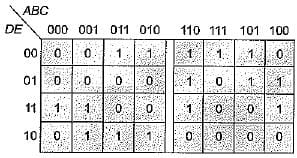Detailed Solution for Test: Boolean Algebra & Minimization Techniques- 2 - Question 19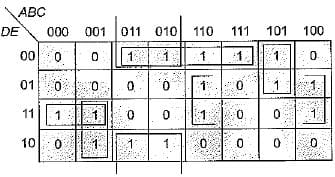From above K-map, output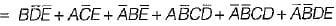Hence, number of minterms in the minimized output are 6.

Test: Boolean Algebra & Minimization Techniques- 2 - Question 20

The simplified expression for the Boolean expression:
f(A, B, C, D) = ∑m (0, 2, 3, 6, 7) + ∑d (8,10,11,15)  is

Detailed Solution for Test: Boolean Algebra & Minimization Techniques- 2 - Question 20

Given:
f = ∑m (0, 2, 3, 6, 7) + ∑d (8, 10, 11, 15)
= πM(1 , 4, 5, 9, 12, 13, 14) + πM(8, 10, 11, 15)
The K-map is shown below: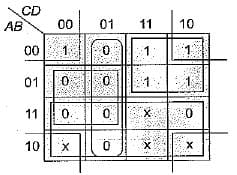Output in SOP form is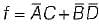.
Output in POS form is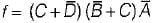Since output can be either in SOP form or in POS form, therefore option (c) is correct.

## Topicwise Question Bank for Electrical Engineering

207 tests
 Use Code STAYHOME200 and get INR 200 additional OFF Use Coupon Code
Information about Test: Boolean Algebra & Minimization Techniques- 2 Page
In this test you can find the Exam questions for Test: Boolean Algebra & Minimization Techniques- 2 solved & explained in the simplest way possible. Besides giving Questions and answers for Test: Boolean Algebra & Minimization Techniques- 2, EduRev gives you an ample number of Online tests for practice

207 tests